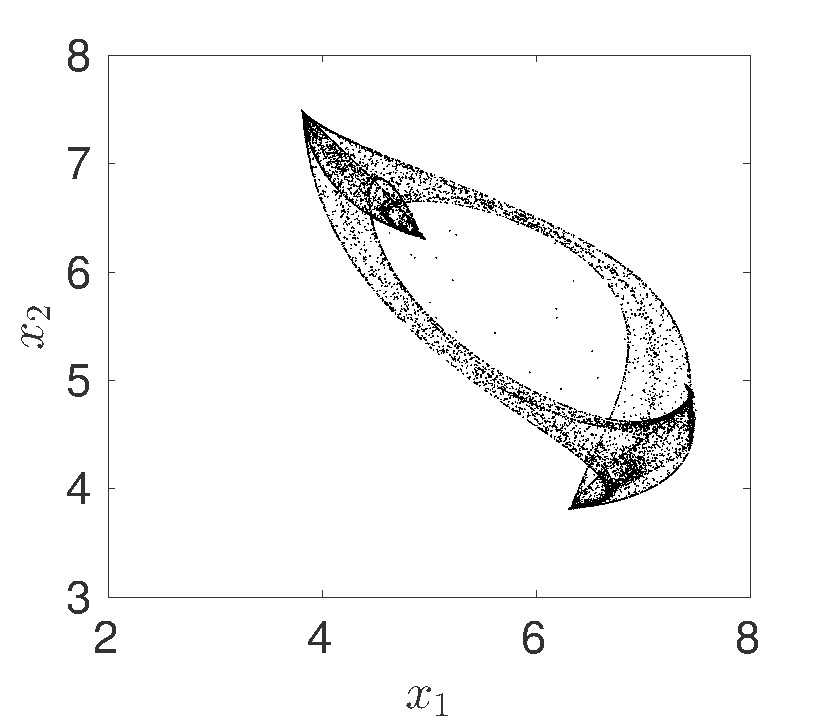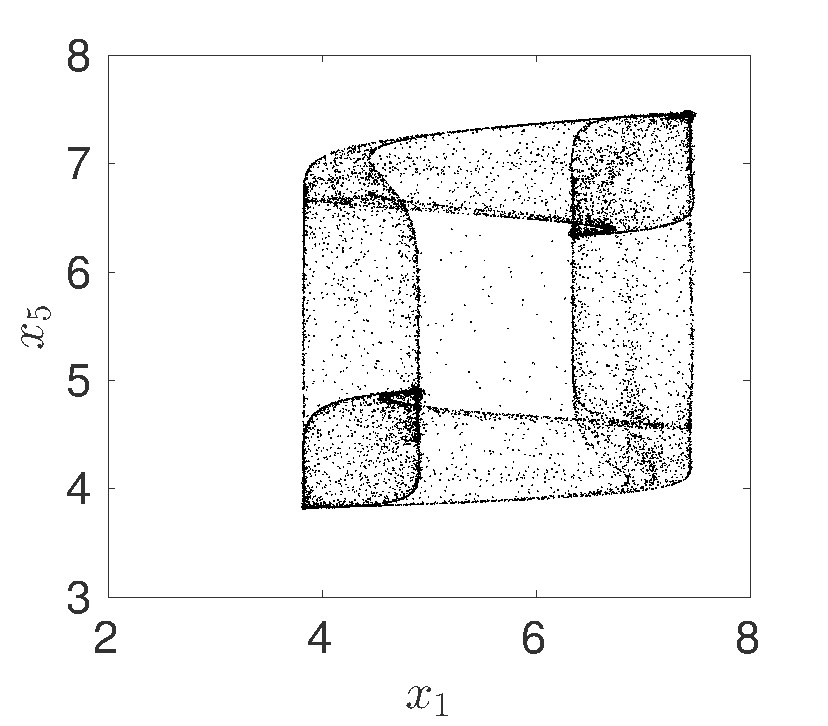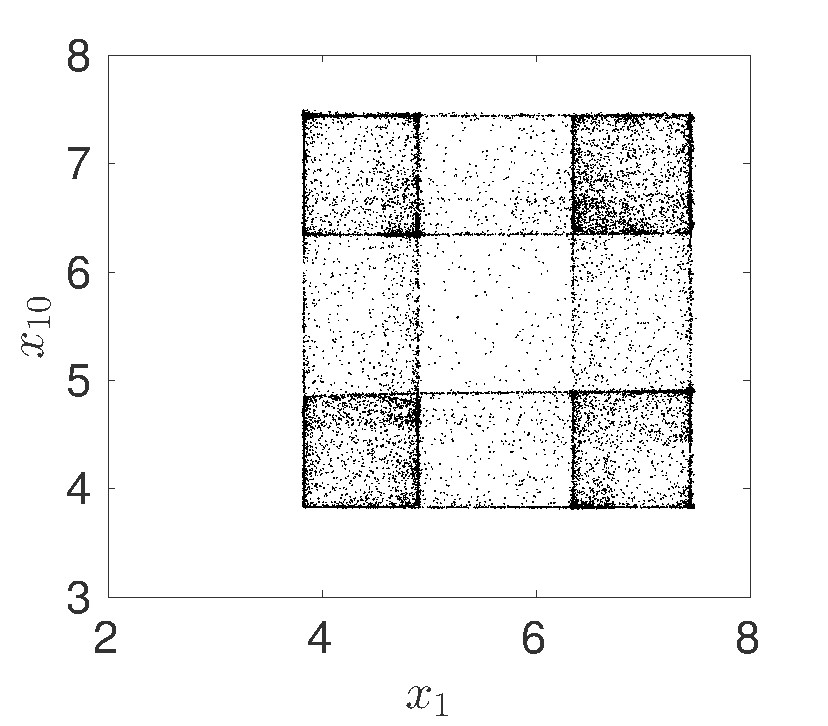Nonlinear Dynamical Systems (AM 114/214)Research Projects          Additional Course Material          Matlab Code

Instructor: Prof. Daniele Venturi (venturi@ucsc.edu)

Teaching Assistants:

• Kenneth Caluya, kcaluya@ucsc.edu

Main Lectures:  Tu/Th 1:30PM -3:05PM (Remote instruction)

Discussion Section: Tuesday 5:20-6:55PMPhysical Sciences, room 114 (hybrid: in-person+remote)

Group tutoring research projects discussion:  Monday 1:20PM-2:25PM (remote)

Office Hours:

• Prof. Daniele Venturi, Friday 10AM-11AM (remote)
• Kenneth Caluya, Tuesday 11AM-12PM (remote)

CANVAS COURSE WEBPAGE: CANVAS

LECTURE NOTES: PDF

Grading policy: See CANVAS course webpage

Purpose of the course: Many complex phenomena in physics, biology, and engineering can be modeled in terms of nonlinear dynamical systems. The purpose of the course is for students to learn how to extract quantitative and qualitative information from such models.

Course content:

• One-Dimensional Dynamical Systems - Geometric approach,  flows on the line, linear and nonlinear stability analysis of fixed points, potential systems, bifurcations (saddle-node, transcritical, pitchfork), normal forms, forward and inverse flow maps.
• Two-Dimensional Dynamical Systems - Geometric approach, existence and uniqueness of solutions, linear systems (analytical solution, classification of fixed points), equilibria in nonlinear systems, Hartman-Grobman theorem, center manifolds, conservative systems, reversible systems, index theory, limit cycles (Liénard systems, criteria to rule out closed orbits), Poincaré-Bendixon theorem,  Poincare maps, linear stability analysis of periodic orbits, bifurcations (normal form of co-dimension 1 bifurcations, heteroclinic and homoclinic bifurcations, Hopf bifurcations, global bifurcations of cycles).
• High-Dimensional Dynamical Systems - Existence and uniqueness of solutions, flow maps, linear systems, Liouville theorem, probability density function equations, Lagrangian and Hamiltonian dynamics, fixed points and stability analysis, center manifolds, bifurcation analysis of equilibria, Hopf bifurcation, Lorenz equations (symmetry, volume contraction, stability analysis of fixed points, trapping region, limit cycles, bifurcation analysis, transient chaos, strange attractor), Lyapunov exponents.
• Discrete Dynamical Systems - Examples (Neural networks, Poincaré map, numerical methods for ODEs, Henon map, Newton's method), n-dimensional maps (fixed points, linear stability analysis), one-dimensional maps (cobwebs, logistic map, tent map), analysis of the logistic map, Lyapunov exponents.

Textbook: Steven H. Strogatz, ``Nonlinear Dynamics and Chaos'', CRC Press, 2018.Mitchal Dichter, ``Student solutions manual for nonlinear dynamics and chaos second edition'', CRC press 2019Other recommended textbooks

• L. Perko, ``Differential equations and dynamical systems'', Springer
• R. C. Robinson, ``An introduction to dynamical systems'', American Mathematical Society.
• S. Wiggins, ``Introduction to applied nonlinear dynamical systems'', Springer.
• M. W. Hirsch, S. Smale and R. L. Devaney, ``Differential equations, dynamical systems, and introdution to chaos'', Academic Press
• Y. A. Kuznetsov, ``Elements of applied bifurcation theory'', Springer (PDF)
• S. N. Rasband, ``Chaotic dynamics of nonlinear systems'', Dover.
• J. Guckenheimer and P. Holmes, ``Nonlinear oscillations, dynamical systems and bifurcations of vector fields'', Springer.